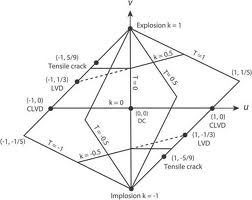## Lune Method Assignment Help

Assignment Help: >> Development of a Sphere - Lune Method

Lune Method:

A sphere may be divided into 12 lunes, one of that is displayed in the front view. The semi-circle qr is the top view of the centre line of that lune. Such length of the line is equal to the length of the arc qr and its maximum width is equal to gh.Divide the semi-circle into a number of equal parts, as like eight and project the division points on the front view to the points 1, 2. Along with q like the centre and the radii equal to q´ 1´, q´ 2? and q 3, draw arcs ab, cd, and ef that will show the widths of the lune on points 1 and 7, 2 and 6, 3 and 5 respectively.

Sketch a line QR equal to the length of the arc qr as with stepping off eight divisions, all equal to the chord-length q1.

Sketch a perpendicular at all division-points and create AB and MN equal to ab at points 1 and 7, CD as well as KL equal to cd at point 2 and 6. Sketches smooth curves by point Q, A, C. The figure hence obtained will be the approximate development of one-twelfth (1/12th) of the surface of the sphere.